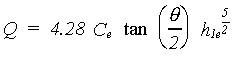USBR Water Measurement Manual

CHAPTER 7 - WEIRS

Section 7:  V-Notch Weirs of Any Angle

7. V-Notch Weirs of Any Angle

The Kindsvater-Shen relationship can be used for fully contracted notches of any angle between 25 degrees and 100 degrees (Kulin and Compton, 1975). The equation which includes the angleas a variable is written as:(7-3)

where:

Q = discharge over weir in ft3/s

Ce = effective discharge coefficient

h1 = head on the weir in ft

h1e = h1 + kh= angle of V-notch

The head correction factor, kh, is a function of(figure 7-6a). However, for fully contracted traditional 90-degree V-notch weirs, equation 7-6 and the rating table discussed later produce comparable accuracy.

 Figure 7-6a -- Head correction factor, kh, for V-notches of any angle (courtesy of National Bureau of Standards, Kulin et al. ).
 Figure 7-6b -- Effective coefficient, Ce, for fully contracted V-notches of any angle (courtesy of National Bureau of Standards, Kulin et al. ).

For fully contracted V-notch weirs, the value of kh is related toas given on figure 7-6a, and values of Ce are read from figure 7-6b. Partially contracted 90-degree V-notches only can be rated using figure 7-7 to obtain Ce values. The calibration relationships were developed with rectangular approach flow and head measurement sections for these weirs. For applications with other flow section shapes, the average width of the flow section for each h1 is used as B to determine coefficients.

 Figure 7-7 -- Effective coefficient, Ce, for partially contracted 90-degree V-notches (courtesy of National Bureau of Standards, Kulin et al. ).

Bos (1989) and International Organization for Standardization (1983) explain and define limits basic to the use of these figures. Precautions and restrictions concerning the use of V-notch weirs are as follows:

(a) V-notch weirs should not be designed beyond the range of the parameters plotted on figures 7-6 and 7-7. Only the 90-degree V-notch weir can be made partially contracted through the use of figure 7-7.

(b) The water surface downstream from the weir should always remain at least 0.2 ft below the notch. Lower discharge readings should be rejected if the contraction is not springing underneath for the entire nappe length.

(c) The measuring head should be greater than 0.2 ft because precision of head measurement error is large relative to smaller head depths, and the nappe may cling to the weir plate.

(d) For the fully contracted V-notch, the maximum measuring head should be less than 1.25 ft.

(e) For the partially contracted V-notch, the maximum head should be less than 2 ft.

(f) For fully contracted V-notches, the h1/B ratio should be equal to or less than 0.2.

(g) For the partially contracted 90-degree notch, h1/B should be equal to or less than 0.4.

(h) The average width of the approach channel, B, should always be greater than 3 ft for the fully contracted V-notch.

(i) For the partially contracted 90-degree V-notch, the approach channel width should be greater than 2 ft.

(j) The V-notch of the weir should always be located at least 1.5 ft above the invert of the weir pool for fully contracted weirs.

(k) Only the 90-degree V-notch can be partially contracted, but the point of the notch must be located at least 4 in from the channel invert.

(l) All the requirements in section 5 apply.

(m) All the approach flow conditions in chapter 2 apply.

Original source:  USBR Water Measurement Manual, Chapter 7, Section 7